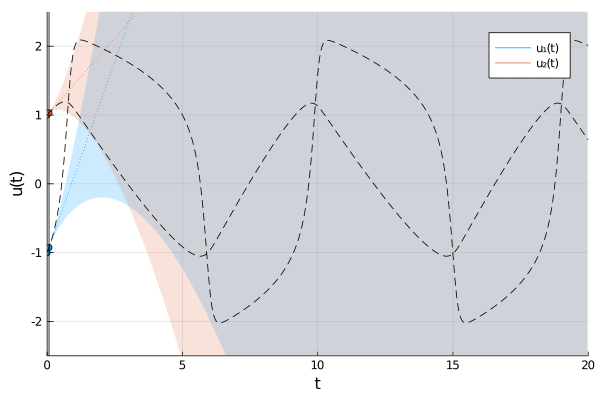ProbNumDiffEq.jl

Probabilistic numerical methods for solving differential equations.ProbNumDiffEq.jl is a library for probabilistic numerical methods for solving differential equations. It provides drop-in replacements for classic ODE solvers from DifferentialEquations.jl by extending OrdinaryDiffEq.jl.

Installation

The package can be installed directly with the Julia package manager:

Tutorials

... more to come!

Gaussian ODE Filters:

• N. Bosch, P. Hennig, F. Tronarp: Calibrated Adaptive Probabilistic ODE Solvers (2021)
• F. Tronarp, S. Särkkä, and P. Hennig: Bayesian ODE Solvers: The Maximum A Posteriori Estimate (2021)
• N. Krämer, P. Hennig: Stable Implementation of Probabilistic ODE Solvers (2020)
• H. Kersting, T. J. Sullivan, and P. Hennig: Convergence Rates of Gaussian Ode Filters (2020)
• F. Tronarp, H. Kersting, S. Särkkä, and P. Hennig: Probabilistic Solutions To Ordinary Differential Equations As Non-Linear Bayesian Filtering: A New Perspective (2019)
• M. Schober, S. Särkkä, and P. Hennig: A Probabilistic Model for the Numerical Solution of Initial Value Problems (2018)

Probabilistic Numerics:

• ProbNum is a Python package for probabilistic numerics. It contains much of the functionality of this package, as well as many other implementations of probabilstic numerical methods.
• http://probabilistic-numerics.org/
• C. J. Oates and T. J. Sullivan: A modern retrospective on probabilistic numerics (2019)
• P. Hennig, M. A. Osborne, and M. Girolami: Probabilistic numerics and uncertainty in computations (2015)